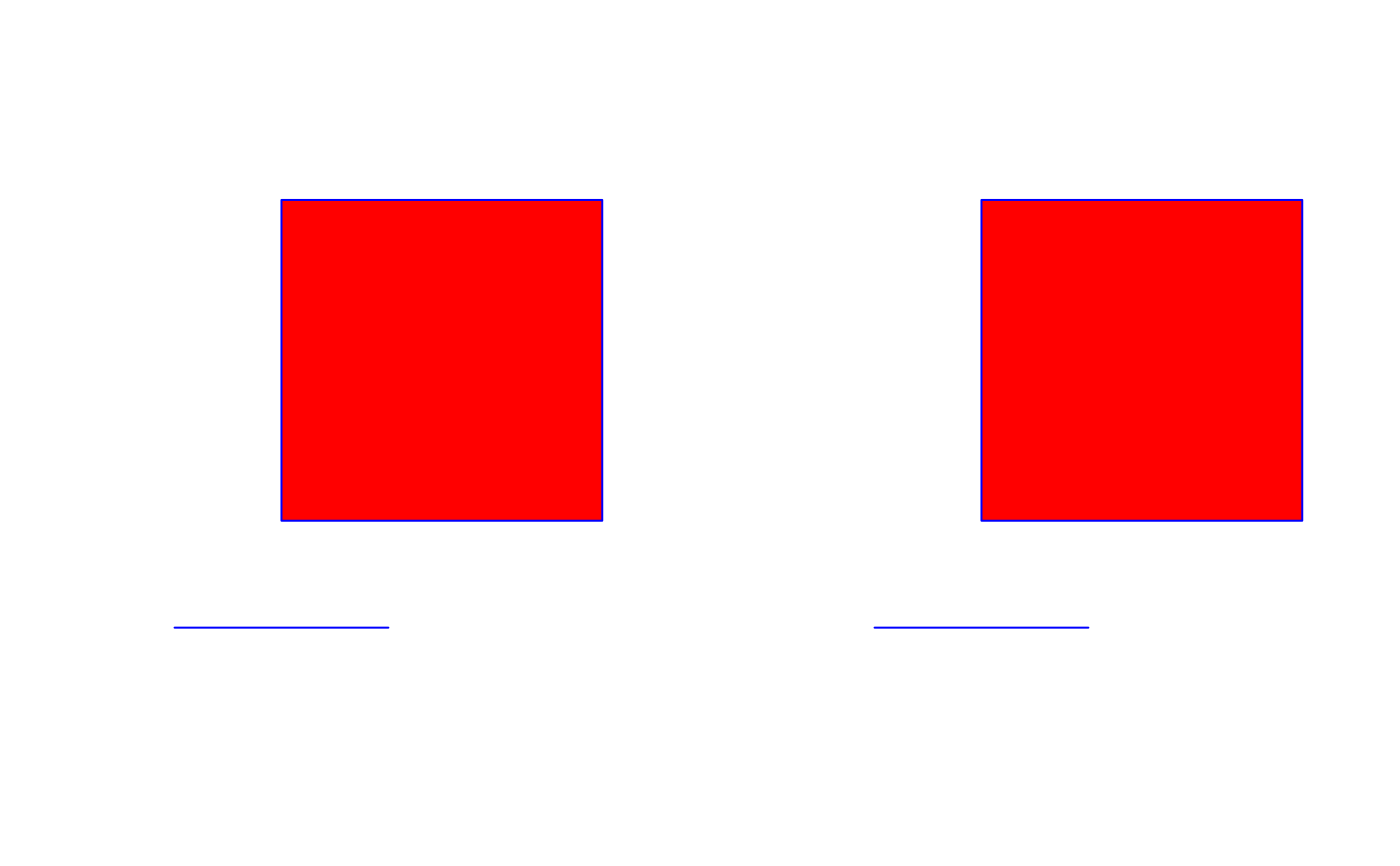How to plot polygons with holes - holes are encoded by coordinates going anticlockwise, and overplotting is avoided by re-ordering the order in which polygons are plotted.

This example is retained for historical interest only, other solutions are present in the sp package.

data(gpcholes)

## Details

"Date: Tue, 11 May 2004 12:54:20 +0900 From: Hisaji ONO To: r-help

I've tried to create a polygon with one hole by gpclib using following example script.

holepoly <- read.polyfile(system.file("poly-ex/hole-poly.txt", package="gpclib"), nohole = FALSE) area.poly(holepoly) plot(holepoly,poly.args=list(col="red",border="blue"))

And I noticed plot function couldn't draw polygons with holes correctly.

Does anyone know how to solve this situation?"

*(h1pl has reversed the y component of polygon 1, to make its ring direction clockwise, h2pl reverses the order of the two polygons in holepoly1@pts)*

## Source

Data file included in "gpclib" package.

## Examples

data(gpcholes)
opar <- par(mfrow=c(1,2))
plot(SpatialPolygons(list(h2pl)), col="red", pbg="white", border="blue")
plot(SpatialPolygons(list(h1pl)), col="red", pbg="white", border="blue")par(opar)